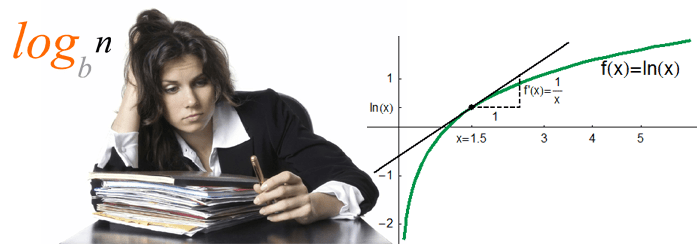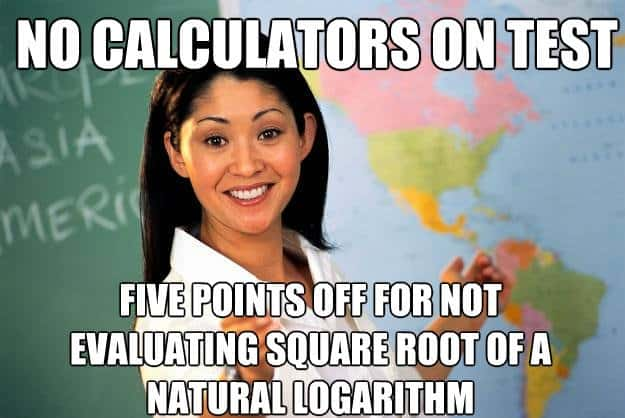# Condensing Logarithmic ExpressionsIf you’re dealing with logarithms, which is not a rare case when you take math classes, the important task is to simplify given expression, reduce number of logarithms, etc. Let’s consider the following problem. We’re required to condense the following expression into single logarithm: $\ln{26}-\ln{23}+2\ln{\sqrt{28}}$

Obviously, we could have taken a calculator to evaluate this right away, yet we’ll do it precisely, you watch. To solve this problem, we need to recall a few formulas or rules, for logarithms.

By the way, check video-tutorial on our channel:

The first formula we’ll use is the following:

$\log_a{x}+\log_a{y}=\log_a{xy}$

This is called the Product Rule for logarithms. The next formula we’ll apply:

$\log_a{x}-\log_a{y}=\log_a{\frac{x}{y}}$

As you may have guessed, it’s the Quotient Rule. What’s important about these two formulas is that the base of all the logarithms is the same. Having logarithms with different bases, you are not allowed to apply these formulas.

Next comes the Power Rule:

$\log_a{x^b}=b \log_a{x}$

It also works reversed:

$b\log_a=\log_a{x^b}$

Now let’s get to our problem. Notice that we have only natural logarithms here, which means they all have the same base $e=2.71828..$. Thus, we can apply the aforementioned formulas:

\begin{aligned}\ln{26}-\ln{23}+2\ln{\sqrt{28}}=\ln{\frac{26}{23}}+2\ln{28^{1/2}}=\ln{\frac{26}{23}}+\ln{(28^{1/2})^2}=&\\ \ln{\frac{26}{23}}+\ln{28}=\ln{\frac{26\cdot 28}{23}}=\ln{\frac{728}{23}}\end{aligned}

That’s it. If you still have questions about logarithms or other math stuff, don’t hesitate to ask!2 Shares
Filed under Math.